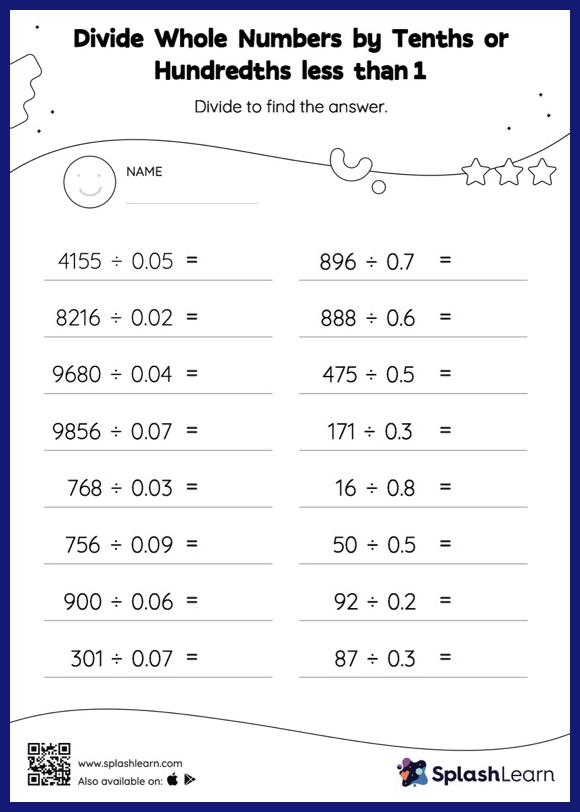# Divide Whole Numbers by Tenths or Hundredths less than 1 without Remainder: Horizontal Division Worksheet

Home > Divide Whole Numbers by Tenths or Hundredths less than 1 without Remainder: Horizontal DivisionThis divide whole numbers by tenths or hundredths less than 1 without remainder worksheet consists of tasks to help your young mathematician develop fluency with division. Students divide a whole number by a decimal without taking the decimal point into account, and then they move each digit of the quotient to the left depending on how many decimal digits there are in the divisor. Divide whole numbers by tenths or hundredths less than 1 without remainder worksheet provides plenty of practice with this concept.